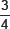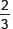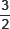# Constructions-Sample Questions

Class IX Math
Sample paper for Construction
1.   Construct an isosceles triangle whose base is 9 cm and altitude is 8 cm. Then construct another triangle whose sides areof the corresponding sides of the first isosceles triangle.
2.   Draw a line segment AB of length 7 cm. Taking A as centre draw a circle of radius 3 cm and taking B as centre, draw another circle of radius 2.5 cm. Construct tangents to each circle from the centre of the other circle.
3.   Construct a ΔABC in which BC = 6.5 cm, AB = 4.5 cm and ∠ABC = 60°. Construct a triangle similar to this triangle whose sides areof the corresponding sides of the ΔABC.
4.   Draw a right triangle in which sides (other than hypotenuse) are of lengths 8 cm and 6 cm. Then construct another triangle whose sides aretimes the corresponding sides of the first triangle.
5.   Construct a triangle with sides 4 cm, 5 cm and 7 cm. Then construct a triangle similar to it whose sides areof the corresponding sides of the given triangle.
6.   Construct a ΔABC in which AB = 6.5 cm, ∠B = 60° and BC = 5.5 cm. Also construct a triangle AB’C’ similar to ΔABC whose each side istimes the corresponding side of the ΔABC.
7.   Draw a ΔABC with side BC = 6 cm, AB = 5 cm and ∠ABC = 60°. Construct ΔAB’C’ similar to ΔABC such that sides of ΔAB’C” areof the corresponding sides of ΔABC.
8.   Construct a triangle whose perimeter is 13.5 cm and the ratio of the three sides is 2 : 3 : 4.